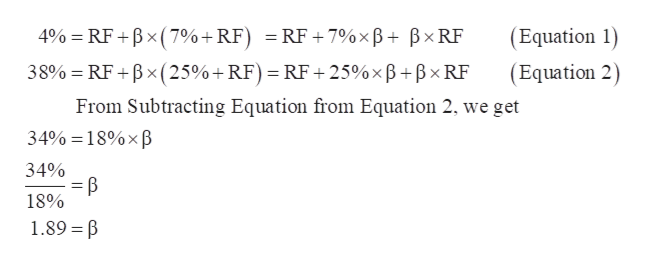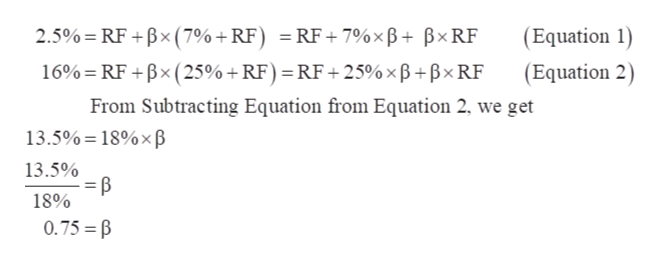# Consider the following table, which gives a security analyst's expected return on two stocks for two particular market returns:Market returnAggressive StockDefensive Stock7%4%2.5%253816If the T-bill rate is 3% and the market return is equally likely to be 7% or 25%, what are the alphas of the two stocks?

Question
96 views

Consider the following table, which gives a security analyst's expected return on two stocks for two particular market returns:

 Market return Aggressive Stock Defensive Stock 7% 4% 2.5% 25 38 16

If the T-bill rate is 3% and the market return is equally likely to be 7% or 25%, what are the alphas of the two stocks?

check_circle

star
star
star
star
star
1 Rating
Step 1

For the Calculation of Alpha, first we have to calculate Beta of each stocks and Beta can be calculated by using this formula:

Expected Return of Stock = Risk-Free Rate (RF) + Beta (β) × (Market Return - Risk-Free Rate (RF))

Calculation of Beta (β) of Aggressive Stock:help_outlineImage Transcriptionclose4%=RF+Bx (7%+RF) = RF +7%xB+ BXRF (Equation 1) 38% RF+Bx (25%+ RF) = RF +25% x B+BXRF (Equation 2) From Subtracting Equation from Equation 2, we get 34%=18%xB 34% 18% 1.89 =B fullscreen
Step 2

Calculation of Beta (β) of Defensive Stock:help_outlineImage Transcriptionclose= RF 2.5%=RF Bx ( 7% + RF) 7%xB+ BXRF (Equation 1) (Equation 2) 16%=RF +Bx (25% + RF) = RF+ 25% x B+Bx RF From Subtracting Equation from Equation 2, we get 13.5%=18%xB 13.5% 18% 0.75 B fullscreen
Step 3

Beta of Aggressive Stock (β) = 1.89

T-Bill Rate or Risk-Free Rate (RF) = 3%

Market Return (MR) = (7% + 25%) ÷ 2 = 16%

Expected ...

### Want to see the full answer?

See Solution

#### Want to see this answer and more?

Solutions are written by subject experts who are available 24/7. Questions are typically answered within 1 hour.*

See Solution
*Response times may vary by subject and question.
Tagged in